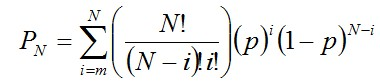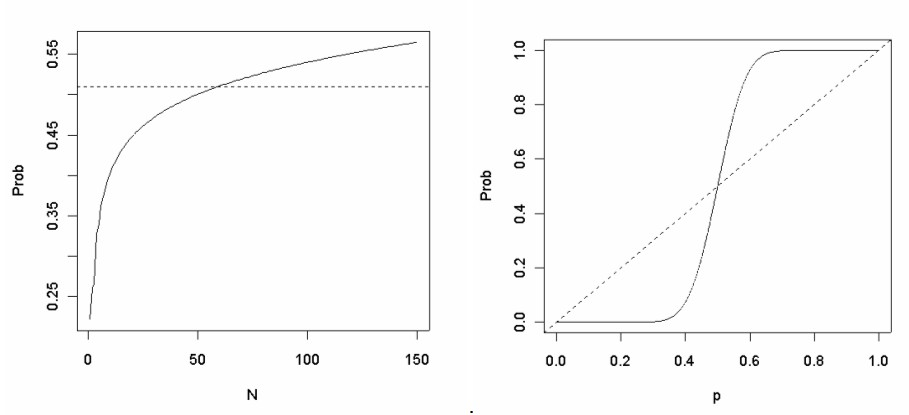# Condorcet's Jury Theorem

Statistical Theory, Socio-political Issues, Democracy

Condorcet's jury theorem calculates the probability, PN ,  that a jury gives the correct answer, given:
• N = the number of jurors
• p = the probability of an individual juror being right
• m = the number of jurors required for a majority

Condorcet’s jury theorem in its simplest form has the following formula:Condorcet’s jury theorem is based around the cumulative binomial distribution.

Condorcet’s jury theorem assumes that all jurors are independent and with the same probability of being right.  Condorcet’s jury theorem can apply to voting theory, given the assumption that there is a 'correct' or 'best' candidate.  When this is done, jurors are replaced with voters.The graph on the left shows PN versus N, with p fixed at 0.51 and m chosen to be N/2 + 1.  The dashed line is horizontal at 0.51.  Once the electorate size gets large enough, as long as people are right more often than they are wrong, the probability that the voting outcome is right is greater than an individual’s probability of being right.

The graph on the right shows PN versus p, with N fixed at 51 and m fixed at 26.  The dashed line is where PN = p.  The graph shows that for a sufficiently large enough electorate, if p  0.5 then PN p and if p 0.5 then PN  p.

The graphs suggest that when an electorate is large enough, and individuals in that electorate are right more often than they are wrong, elections would act as a ‘truth tracker’.

The limitations of the theorem include:

The independence assumption.  Voters can influence each other, leading to within-group correlations.  These groups could be tiny, such as a family unit or a circle of friends; or much larger, such as a religious group, socio-economic class or geographic area.

The equal probabilities assumption.  Some voters would be more informed than others.  Some voters would have better judgement than others.  Most individuals’ voting decisions wouldn’t be based on chance, and they would form a firm opinion of who to vote for.

The notion of the ‘best’ candidate.  Government policy is often a matter of tradeoffs, rather than optimisation.  Even if voters are well informed, they might not necessarily vote the same way because of different values, different personal circumstances and different priorities when it comes to individual interest versus collective interest.

Vote splitting.  When there are more than two candidates, the vote splitting problem would occur.  For example, let’s say there are three candidates, A, B and C with 40%, 35% and 25% of the votes respectively.  Let’s say that all C voters prefer candidate B over A.  In this situation, candidate A wins despite the majority of voters preferring candidate B.  When there are more than two candidates, people tend to vote for the lesser of the two greatest evils most likely to win, rather than their most preferred candidate.  Condorcet also devised a voting method that overcomes this problem.  It involves ranking the candidates in order of preference, rather than giving a single tick to a candidate.